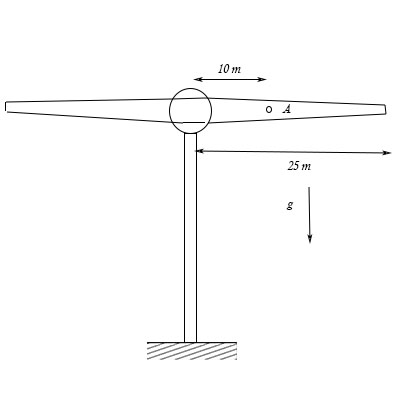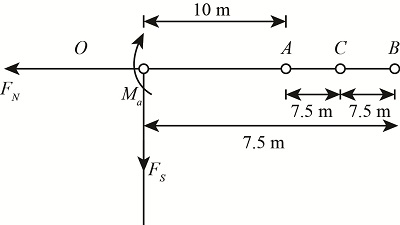# The lightweight turbine consist of a rotor with horizontal axis powered form a torque applied at...

## Question:

The lightweight turbine consist of a rotor with horizontal axis powered form a torque applied at it center. At the instant the rotor has a clockwise angular velocity of {eq}10 rad/s {/eq} and a clockwise angular acceleration of {eq}8 rad/s^2 {/eq}. Determine the internal normal force shear force, and moment at a section through {eq}A {/eq}. Assume the rotor is a {eq}50 m {/eq} long slender rod, having a mass of {eq}2 kg/m. {/eq}## Moment

The term moment is determined as the product of force and the distance where the force is applied. The moment is analogous of the torque. The moment is also defined as a measure by virtue of which the body rotates when applied on it.

## Answer and Explanation:

Given Data

• The angular velocity of the rotor is: {eq}{\omega _{ro}} = 10\;{\rm{rad/s}} {/eq}
• The angular acceleration of the rotor is: {eq}{\alpha _{ro}} = 8\;{\rm{rad/s}} {/eq}
• The length of the rod is: {eq}{L_r} = 50\;{\rm{m}} {/eq}
• The mass of the rotor is: {eq}{m_r} = {\rm{2}}\;{\rm{kg/m}} {/eq}
• The length OA of rotor is: {eq}OA = 10\;{\rm{m}} {/eq}

Free body diagram of the rotorThe expression for the center of radius,

{eq}{R_c} = \left( {\dfrac{{\dfrac{{{L_r}}}{2} - OA}}{2}} \right) + OA {/eq}

Substitute the given data in above equation,

{eq}\begin{align*} {R_c} &= \left( {\dfrac{{\dfrac{{50}}{2} - 10}}{2}} \right) + 10\\ &= 17.5\;{\rm{m}} \end{align*} {/eq}

The expression for the normal force taking force equilibrium in horizontal direction,

{eq}\begin{align*} {F_N} - {F_C} &= 0\\ {F_N} &= \left( {{m_r} \times \left( {\dfrac{{{L_r}}}{2} - OA} \right)} \right){\left( {{\omega _{ro}}} \right)^2}{R_c} \end{align*} {/eq}

Here {eq}{F_N} {/eq} is the normal force and {eq}{F_C} {/eq}is the centrifugal force,

Substitute the given data in above equation,

{eq}\begin{align*} {F_N} &= \left( {2 \times \left( {\dfrac{{50}}{2} - 10} \right)} \right){\left( {10} \right)^2}\left( {17.5} \right)\\ {F_N} &= 52500\;{\rm{N}} \end{align*} {/eq}

Thus the normal force at A is{eq}52500\;{\rm{N}} {/eq}

The expression for the shear force at A is,

{eq}{F_s} = m{\alpha _{ro}}{R_c} - mg {/eq}

Substitute the given data in above equation,

{eq}\begin{align*} {F_s} &= \left( {2 \times \left( {\dfrac{{50}}{2} - 10} \right)} \right)\left( {8 \times 17.5 - \left( {2 \times \left( {\dfrac{{50}}{2} - 10} \right)} \right) \times 9.81} \right)\\ &= 3905.7\;{\rm{N}} \end{align*} {/eq}

Thus the shear force at A is {eq}3905.7\;{\rm{N}} {/eq}

The expression for the moment at A,

{eq}\begin{align*} {M_a} &= {I_{ro}}\alpha + m \times \alpha \times {R_c} - \left( {mg \times \left( {{R_c} - OA} \right)} \right)\\ &= \left( {\dfrac{m}{{12}} \times {{\left( {\dfrac{{{L_r}}}{2} - OA} \right)}^2}} \right) + m \times \alpha \times {R_c} - \left( {mg \times \left( {{R_c} - OA} \right)} \right) \end{align*} {/eq}

Substitute the given data in above equation,

{eq}\begin{align*} {M_a} &= \left( {\left( {\dfrac{2}{{12}} \times \left( {\dfrac{{50}}{2} - 10} \right)} \right) \times {{\left( {\dfrac{{50}}{2} - 10} \right)}^2}} \right) + \left( {2 \times \left( {\dfrac{{50}}{2} - 10} \right)} \right) \times 8 \times 17.5 - \left( {\left( {2 \times \left( {\dfrac{{50}}{2} - 10} \right)} \right)9.81 \times \left( {17.5 - 10} \right)} \right)\\ &= 2555.5\;{\rm{N}} \cdot {\rm{m}} \end{align*} {/eq}

Thus the moment at A is {eq}2555.5\;{\rm{N}} \cdot {\rm{m}} {/eq}

#### Learn more about this topic:Torque in Physics: Equation, Examples & Problems

from Physics: Middle School

Chapter 3 / Lesson 13
43K